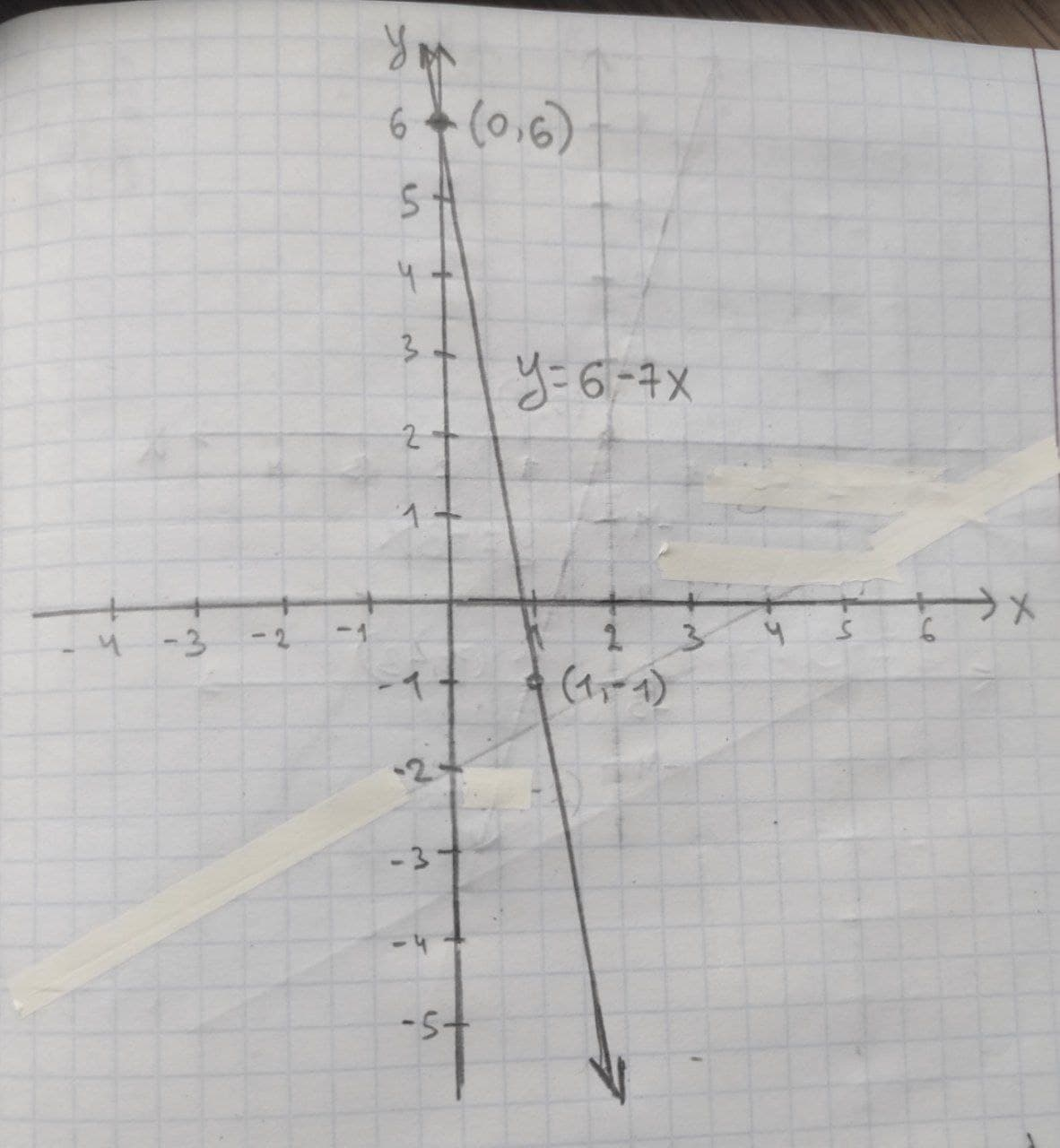Given linear equation y = 6 − 7x a. find the y-intercept and slope. b. determine whether the line slopes upward, slopes downward, or is horizontal, without graphing the equation. c. use two points to graph the equation.Harlen Pritchard 2021-01-04 Answered
Given linear equation y = 6 − 7x
a. find the y-intercept and slope.
b. determine whether the line slopes upward, slopes downward, or is horizontal, without graphing the equation.
c. use two points to graph the equation.

• Questions are typically answered in as fast as 30 minutes

Solve your problem for the price of one coffee

• Math expert for every subject
• Pay only if we can solve itArham Warner

(a) The linear equation is y=6−7x.
In a linear equation $$\displaystyle{y}={b}_{{0}}+{b}_{{1}}{x}$$ the constant $$\displaystyle{b}_{{1}}$$ be the slope and $$\displaystyle{b}_{{0}}$$ be the y-intercept form and x is the independent variable and y is the independent variable.
Comparing the given equation with the general form of linear equation the slope of the equation is –7 and the y-intercept is 6.
Thus, the slope of the linear equation is $$\displaystyle{b}_{{1}}=−{7}$$ and the y-intercept is $$\displaystyle{b}_{{0}}={6}$$.
(b) In a linear equation $$\displaystyle{y}={b}_{{0}}+{b}_{{1}}{x}$$ the constant $$\displaystyle{b}_{{1}}$$ be the slope and $$\displaystyle{b}_{{0}}$$ be the y-intercept form and x is the independent variable and y is the independent variable.
It is known that, the slope of the linear equation $$\displaystyle{y}={b}_{{0}}+{b}_{{1}}{x}$$ is upward if $$\displaystyle{b}_{{1}}{>}{0}$$, the slope of the linear equation $$\displaystyle{y}={b}_{{0}}+{b}_{{1}}{x}{y}={b}_{{0}}+{b}_{{1}}{x}$$ is downward if $$\displaystyle{b}_{{1}}{<}{0}$$ and the slope of the linear equation $$\displaystyle{y}={b}_{{0}}+{b}_{{1}}{x}$$ is horizontal if $$\displaystyle{b}_{{1}}={0}.$$
Thus, in the given equation $$\displaystyle{y}={6}−{7}{x},{b}_{{1}}=−{7}{<}{0}.$$
Thus, the slope is downward.
(c) The linear equation is y=6−7x.
Graph the line:
The two points $$\displaystyle{\left({x}_{{1}}, {y}_{{1}}\right)}{\quad\text{and}\quad}{\left({x}_{{2}}, {y}_{{2}}\right)}$$ on the given line are obtained:
If x = 0,
$$y=6+(−7 \times 0)$$
y=6
Thus, one point on the line is $$\displaystyle{\left({x}_{{1}}, {y}_{{1}}\right)}={\left({0},{6}\right)}.$$
If x = 1,
$$y=6+(−7 \times 1)$$
y=−1
Thus, the second point on the line is $$\displaystyle{\left({x}_{{2}}, {y}_{{2}}\right)}={\left({1},−{1}\right)}.$$